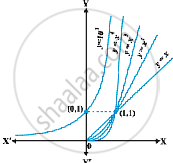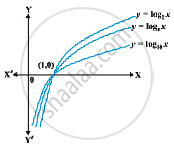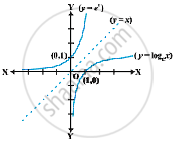# Exponential and Logarithmic Functions

#### definition

The exponential function with positive base b > 1 is the function y = f(x) = bx
The graph of y = 10x is given in the Fig.It is advised that the reader plots this graph for particular values of b like 2, 3 and 4. Following are some of the salient features of the exponential functions:
(1) Domain of the exponential function is R, the set of all real numbers.
(2) Range of the exponential function is the set of all positive real numbers.
(3) The point (0, 1) is always on the graph of the exponential function (this is a restatement of the fact that b0 = 1 for any real b > 1).
(4) Exponential function is ever increasing; i.e., as we move from left to right, the graph rises above.
(5) For very large negative values of x, the exponential function is very close to 0. In other words, in the second quadrant, the graph approaches x-axis (but never meets it).

Exponential function with base 10 is called the common exponential function.
The sum of the series

1+1/1! + 1/2!+...
is a number between 2 and 3 and is denoted by e. Using this e as the base we obtain an extremely important exponential function y = ex. This is called natural exponential function.

#### definition

Let b > 1 be a real number. Then we say logarithm of a to base b is x if b^x = a. Logarithm of a to base b is denoted by log_b a. Thus logb a = x if bx = a.  Let us work with a few explicit examples to get a feel for this.
A  base b > 1, we may look at logarithm as a function from positive real numbers to all real numbers. This function, called the logarithmic function, is defined by
log_b : R^+ -> R
x -> log_b x = y  if b^y = x
As before if the base b = 10, we say it is common logarithms and if b = e, then we say it is natural logarithms. Often natural logarithm is denoted by ln. In this chapter, log x denotes the logarithm function to base e, i.e., ln x will be written as simply log x. Fig.the plots of logarithm function to base 2, e and 10.
Some of the important observations about the logarithm function to any base b > 1 are listed below:
(1) We cannot make a meaningful definition of logarithm of non-positive numbers and hence the domain of log function is R^+.
(2) The range of log function is the set of all real numbers.
(3) The point (1, 0) is always on the graph of the log function.
(4) The log function is ever increasing, i.e., as we move from left to right the graph rises above.
(5) For x very near to zero, the value of log x can be made lesser than any given real number. In other words in the fourth quadrant the graph approaches y-axis (but never meets it).
(6) The plot of y = ex and y = ln x. It is of interest to observe that the two curves are the mirror images of each other reflected in the line y = x. Fig.Two  properties of ‘log’ functions are proved below:
(1) There is a standard change of base rule to obtain log_a p in terms of log_bp. Let log_a p = α, log_b p = β and log_b a = γ. This means a^α = p, b^β = p and b^γ = a. Substituting the third equation in the first one, we have
(b^γ)^α = b^(γα) = p
Using this in the second equation, we get b^β = p = b^(γα)
which implies β = αβ or α = β/γ . But then
log_a p = (log_bp)/(log_ba)

(2) Another interesting property of the log function is its effect on products. Let log_b pq = α. Then b^α = pq.
If log_b p = β and log_b q = γ, then b^β = p and b^γ = q.
But then b^α = pq = b^βb^γ = b^(β + γ)
which implies α = β + γ, i.e.,
log_b pq = log_b p + log_b q
A particularly interesting and important consequence of this is when p = q.
In this case the above may be rewritten as log_b p^2 = log_b p + log_b p = 2 log p
An easy generalisation of this (left as an exercise!) is
log_b p^n = n log p
for any positive integer n. In fact this is true for any real number n, but we will not attempt to prove this. On the similar lines the reader is invited to verify
log _b  x/y = log_b x - log _b y

If you would like to contribute notes or other learning material, please submit them using the button below.

### Shaalaa.com

Derivative of Exponential Functions [00:27:24]
S
0%